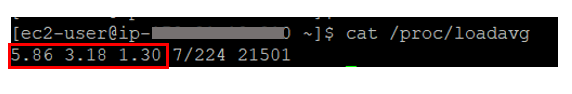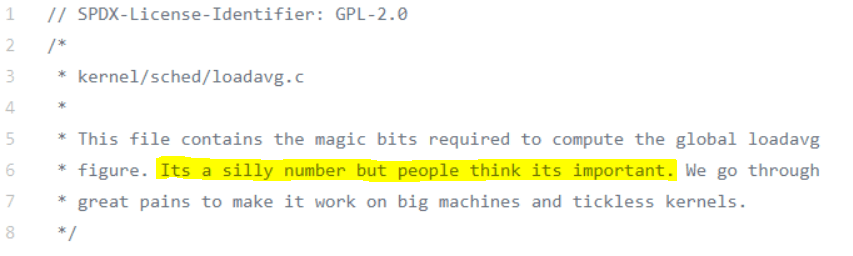##What is Load Average?

In most cases, Load average is reported as an average of 1 minute, 5 minutes and 15 minutes. Please refer to the below screenshot: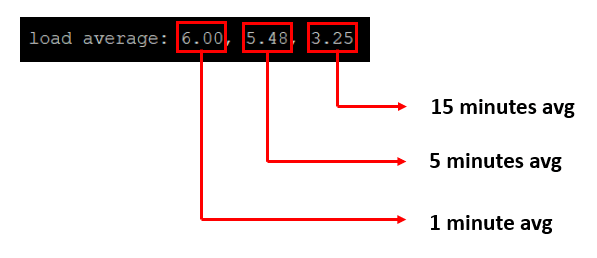Its hard to say whether Load Average is high, normal, or low without knowing the number of CPUs present in the system. You can find out the number of CPUs in the system through one of the approaches given here

1 CPU:

In the above example, we saw 1-minute load average as 6.00. Suppose the above system has just 1 CPU, then it indicates Load Average is quite high on this machine. It means this system is processing 600% load in the last minute. We derive 600% because

= (Load Average / Number of CPUs) x 100

= (6.00 / 1) x 100

= 600%

Thus, the system is 500% overloaded more than the capacity it can handle.

12 CPUs:

Say suppose the above system has 12 CPUs, it means Load Average is normal. Since the 1-minute Load Average is 6.00, it means only 50% of the compute capacity is utilized. We derive 50% because

= (Load Average / Number of CPUs) x 100

= (6.00 / 12) x 100

= 50%

24 CPUs:

Suppose the above system has 24 CPUs, it means Load Average is quite low. Since the 1 minute Load Average is 6.00, it means only 16.66% of the compute capacity is utilized. We derive 16.66% because

= (Load Average / Number of CPUs) x 100

= (6.00 / 24) x 100

= 16.66%

In this scenario, we can fairly conclude that this system is underutilized

What is the use of 1 minute, 5 minutes, 15 minutes Load Average?
Load Average intervals help determine whether the systems demand for compute is increasing over the period or decreasing over time.Look at the above Load Average reporting. You can notice in the above example Load Average in the system has been increasing. i.e., 15 minutes before this systems Load Average was 3.25. 5 minutes before systems Load Average was 5.48, and the last 1-minute Load Average was 6.00. It indicates the demand for compute is increasing on this system.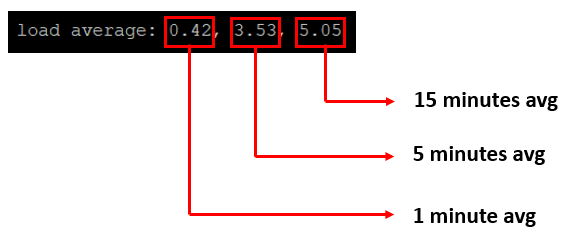Look at the above Load average reporting. You can notice in the above example Load Average in the system has been decreasing. i.e., 15 minutes before this systems Load Average was 5.05. 5 minutes before systems Load Average was 3.53, and the last 1-minute Load Average was 0.42. It indicates the load on this system has been on the decreasing trend.

Does Load Average measure only CPU demand?
It is often told that Load Average only indicates the CPU demand on the system. But its not true. Load Average indicates not only CPU demand but also file I/O demand, network I/O demand, disk I/0 demand, and cycles waiting for locks. Here is an interesting case study we conducted to prove this theory.

Load average is fairly a relative term. What might be a good Load Average for one application can be a bad load average for another application. If you ask me what a rule of thumb is, I would say if Load Average percentage goes beyond 80%, you might want to investigate it.

Load Average can be found from various sources:

a. Unix/Linux command-line tool top reports Load Average in the field as highlighted in the below image.Fig: Load average reported in top command

b. Unix/Linux command-line tool uptime reports Load Average in the field as highlighted in the below image.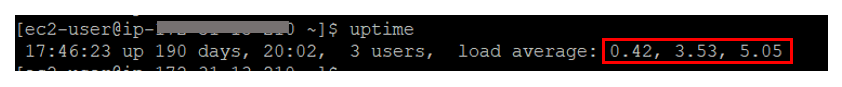Fig: Load average reported in uptime command